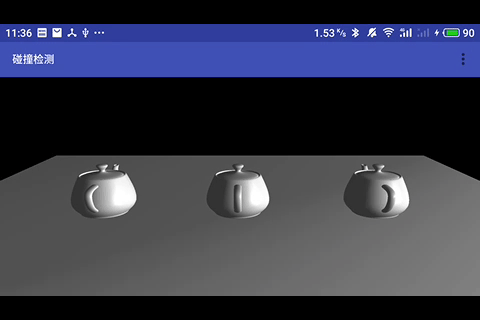# 《OpenGL ES 3.x 游戏开发》碰撞检测之 AABB 包围盒

## 学习笔记内容摘录

Posted by 音视频开发进阶 on Thursday, July 26, 2018

## 碰撞检测实现原理

AABB 包围盒就是采用一个长方体将物体包裹起来，进行两个物体的相交性检测时仅检测物体对应包围盒（包裹物体的长方体）的相交性。\$\$ x_{min} \le x \le x_{max} \$\$ \$\$ y_{min} \le y \le y_{max} \$\$ \$\$ z_{min} \le z \le z_{max} \$\$

\$\$P_{min} = [x_{min} , y_{min} , z_{min}]\$\$ \$\$P_{max} = [x_{max} , y_{max} , z_{max}]\$\$

\$\$c=(P_{min} + P_{max}) / 2\$\$

## AABB 包围盒计算

`````` 1public class Vector3f {
2    float x;//三维变量中的x值
3    float y;//三维变量中的y值
4    float z;//三维变量中的z值
5    public Vector3f(float x,float y,float z)
6    {
7        this.x=x;
8        this.y=y;
9        this.z=z;
10    }
12    {
13        this.x+=temp.x;
14        this.y+=temp.y;
15        this.z+=temp.z;
16    }
17}
``````

`````` 1public class AABBBox {
2    float minX;//x轴最小位置
3    float maxX;//x轴最大位置
4    float minY;//y轴最小位置
5    float maxY;//y轴最大位置
6    float minZ;//z轴最小位置
7    float maxZ;//z轴最大位置
8
9    public AABBBox(float[] vertices)
10    {
11        init();
12        findMinAndMax(vertices);
13    }
14
15    public AABBBox(float minX,float maxX,float minY,float maxY,float minZ,float maxZ)
16    {
17        this.minX=minX;
18        this.maxX=maxX;
19        this.minY=minY;
20        this.maxY=maxY;
21        this.minZ=minZ;
22        this.maxZ=maxZ;
23    }
24    //初始化包围盒的最小以及最大顶点坐标
25    public void init()
26    {
27        minX=Float.POSITIVE_INFINITY;
28        maxX=Float.NEGATIVE_INFINITY;
29        minY=Float.POSITIVE_INFINITY;
30        maxY=Float.NEGATIVE_INFINITY;
31        minZ=Float.POSITIVE_INFINITY;
32        maxZ=Float.NEGATIVE_INFINITY;
33    }
34    //获取包围盒的实际最小以及最大顶点坐标
35    public void findMinAndMax(float[] vertices)
36    {
37        for(int i=0;i<vertices.length/3;i++)
38        {
39            //判断X轴的最小和最大位置
40            if(vertices[i*3]<minX)
41            {
42                minX=vertices[i*3];
43            }
44            if(vertices[i*3]>maxX)
45            {
46                maxX=vertices[i*3];
47            }
48            //判断Y轴的最小和最大位置
49            if(vertices[i*3+1]<minY)
50            {
51                minY=vertices[i*3+1];
52            }
53            if(vertices[i*3+1]>maxY)
54            {
55                maxY=vertices[i*3+1];
56            }
57            //判断Z轴的最小和最大位置
58            if(vertices[i*3+2]<minZ)
59            {
60                minZ=vertices[i*3+2];
61            }
62            if(vertices[i*3+2]>maxZ)
63            {
64                maxZ=vertices[i*3+2];
65            }
66        }
67    }
68    //获得物体平移后的AABB包围盒
69    public AABBBox getCurrAABBBox(Vector3f currPosition)
70    {
71        AABBBox result=new AABBBox
72                (
73                        this.minX+currPosition.x,
74                        this.maxX+currPosition.x,
75                        this.minY+currPosition.y,
76                        this.maxY+currPosition.y,
77                        this.minZ+currPosition.z,
78                        this.maxZ+currPosition.z
79                );
80        return result;
81    }
82}
``````

## AABB 包围盒的碰撞检测`````` 1    public boolean check(RigidBody ra, RigidBody rb)//true为撞上
2    {
3        float[] over = calOverTotal
4                (
5	                // 两个物体的 AABB 包围盒
6                        ra.collObject.getCurrAABBBox(ra.currLocation),
7                        rb.collObject.getCurrAABBBox(rb.currLocation)
8                );
9        // 三个方向的交叠值与设定的阈值进行比较
10        return over > V_UNIT && over > V_UNIT && over > V_UNIT;
11    }
12
13	// 传入两个物体的 AABB 包围盒
14    public float[] calOverTotal(AABBBox a, AABBBox b) {
15        float xOver = calOverOne(a.maxX, a.minX, b.maxX, b.minX);
16        float yOver = calOverOne(a.maxY, a.minY, b.maxY, b.minY);
17        float zOver = calOverOne(a.maxZ, a.minZ, b.maxZ, b.minZ);
18        return new float[]{xOver, yOver, zOver};
19    }
20	// 计算每个轴方向的交叠值
21    public float calOverOne(float amax, float amin, float bmax, float bmin) {
22        float minMax = 0;
23        float maxMin = 0;
24        if (amax < bmax)//a物体在b物体左侧
25        {
26            minMax = amax;
27            maxMin = bmin;
28        } else //a物体在b物体右侧
29        {
30            minMax = bmax;
31            maxMin = amin;
32        }
33
34        if (minMax > maxMin) {
35            return minMax - maxMin;
36        } else {
37            return 0;
38        }
39    }
``````

## 实践RigidBody 代码如下：

`````` 1public class RigidBody {
2    CollisionObject renderObject;//渲染者
3    AABBBox collObject;//碰撞者
4    boolean isStatic;//是否静止的标志位
5    Vector3f currLocation;//位置三维变量
6    Vector3f currV;//速度三维变量
7    final float V_UNIT = 0.02f;//阈值
8
9    public RigidBody(CollisionObject renderObject, boolean isStatic, Vector3f currLocation, Vector3f currV) {
10        this.renderObject = renderObject;
11        collObject = new AABBBox(renderObject.vertices);
12        this.isStatic = isStatic;
13        this.currLocation = currLocation;
14        this.currV = currV;
15    }
16
17    public void drawSelf() {
18        MatrixState.pushMatrix();//保护现场
19        MatrixState.translate(currLocation.x, currLocation.y, currLocation.z);
20        renderObject.drawSelf();//绘制物体
21        MatrixState.popMatrix();//恢复现场
22    }
23
24    public void go(ArrayList<RigidBody> al) {
25        if (isStatic) return;
27        for (int i = 0; i < al.size(); i++) {
28
29            RigidBody rb = al.get(i);
30            if (rb != this) {
31                if (check(this, rb))//检验碰撞
32                {
33                    this.currV.x = -this.currV.x;//哪个方向的有速度，该方向上的速度置反
34                }
35            }
36        }
37    }
38// 省略碰撞检测的代码，在上面已经有了。
39}
``````

``````1    Vector3f currLocation;//位置三维变量
2    Vector3f currV;//速度三维变量
3    // 位置向量加上速度向量，就改变了物体的位置
5    // translate 方法让改变生效
6    MatrixState.translate(currLocation.x, currLocation.y, currLocation.z);
``````

``````1                if (check(this, rb))//检验碰撞，如果发生碰撞了，改变方向
2                {
3                    this.currV.x = -this.currV.x;//哪个方向的有速度，该方向上的速度置反
4                }
``````

`````` 1    var aList = ArrayList<RigidBody>() // 定义刚体集合
2	    // onSurfaceCreated 方法里面添加要绘制的物体，
3        override fun onSurfaceCreated(gl: GL10?, config: EGLConfig?) {
4            aList.add(RigidBody(ch, true, Vector3f(-13f, 0f, 0f), Vector3f(0f, 0f, 0f)))
5            aList.add(RigidBody(ch, true, Vector3f(13f, 0f, 0f), Vector3f(0f, 0f, 0f)))
6            aList.add(RigidBody(ch, false, Vector3f(0f, 0f, 0f), Vector3f(0.1f, 0f, 0f)))
7			// 开启一个线程来改变刚体的移动
9            lgt.start()
10        }
11        // 绘制刚体
12        override fun onDrawFrame(gl: GL10?) {
13            GLES30.glClear(GLES30.GL_DEPTH_BUFFER_BIT or GLES30.GL_COLOR_BUFFER_BIT)
14            for (it in 0 until aList.size) {
15                aList[it].drawSelf()
16            }
17            pm.drawSelf()
18        }
``````

`````` 1public class LovoGoThread extends Thread {
2    ArrayList<RigidBody> al;//控制列表
3    boolean flag = true;//线程控制标志位
5        this.al = al;
6    }
7    public void run() {
8        while (flag) {
9            int size = al.size();
10            for (int i = 0; i < size; i++) {
11                al.get(i).go(al);
12            }
13            try {
14                sleep(20);
15            } catch (Exception e) {
16                e.printStackTrace();
17            }
18        }
19    }
20}
``````

https://github.com/glumes/AndroidOpenGLTutorial

## 注意点

AABB 包围盒的碰撞检测其实还是一种间接检测方案，所以肯定还是会有误差的。

AABB 包围盒对于本身横平竖直的物体在平行于坐标轴摆放的情况下下，计算碰撞检测的误差很小，但对于不规则形状的物体或本身横平竖直的物体在随意倾斜摆放时，计算碰撞检测的误差就会比较大，如下图所示：## 参考

1. 《OpenGL ES 3.x 游戏开发》